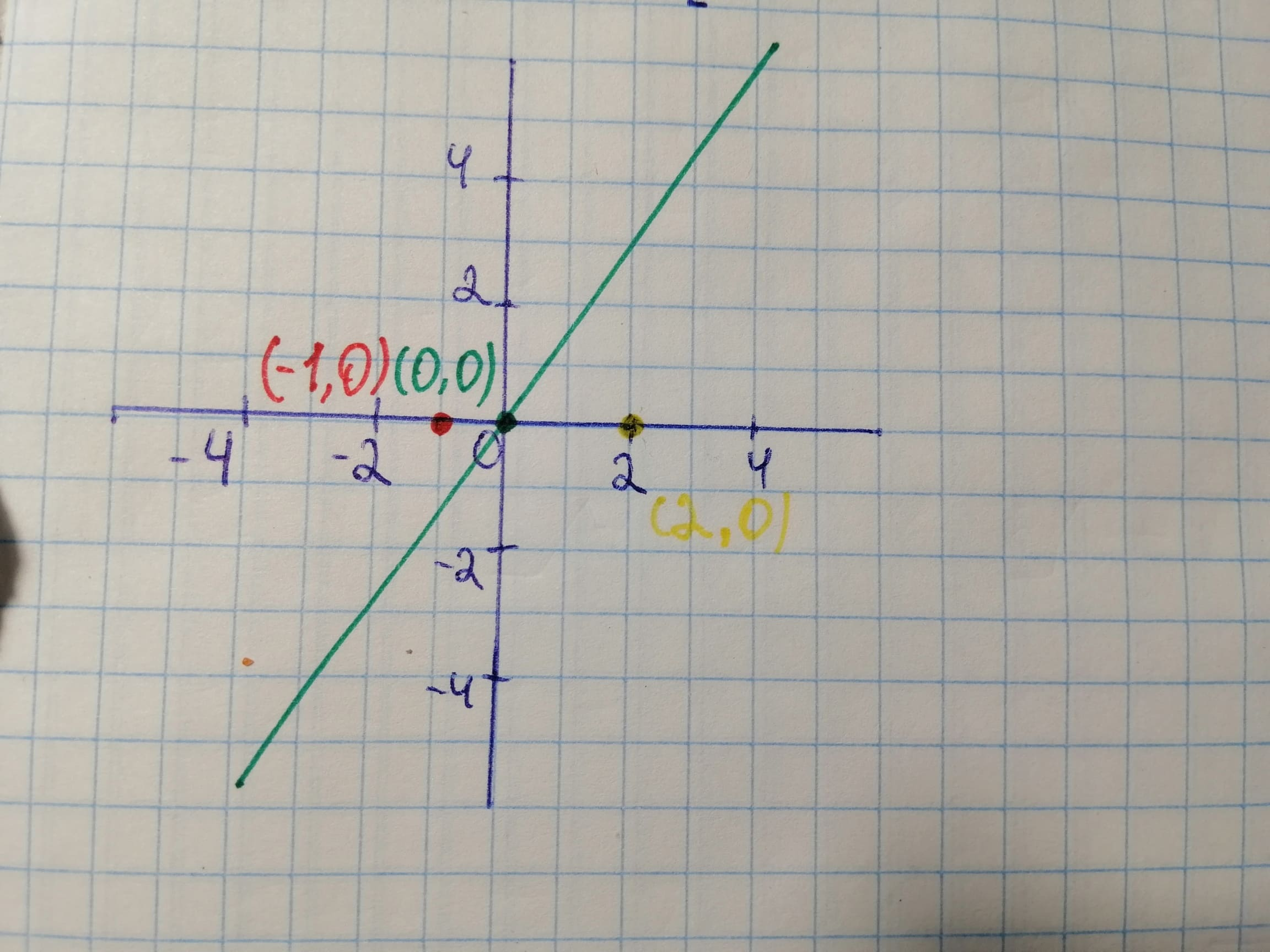# Sketch a graph of y = 2x on [-1, 2] and use geometry to find the exact value of function given int_{-1}^{2} 2x dxFalak Kinney 2021-01-31 Answered
Sketch a graph of $y=2xon\left[-1,2\right]$ and use geometry to find the exact value of function given
You can still ask an expert for help

• Questions are typically answered in as fast as 30 minutes

Solve your problem for the price of one coffee

• Math expert for every subject
• Pay only if we can solve itAamina Herring

Let's sketch the graph of the following function:
$y=2x$
on $\left[-1,2\right]$We can use several methods to find the value of the given expression either by Riemann Sum, or by another sum methods where we need to divide this interval into equal sub-intervals : But we use the easiest method that is we can simply integrate the following expression using the property of integration and then apply the limits:
The given expression is :

Let's integrate the following expression:

$=2\left[\frac{{x}^{2}}{2}{\right]}_{-1}^{2}$
$=\left[{x}^{2}{\right]}_{-1}^{2}$
$=\left[\left(2{\right)}^{2}-\left(-1{\right)}^{2}\right]$
$=\left[4-1\right]=3$ Hence, the value of the given expression is : 3